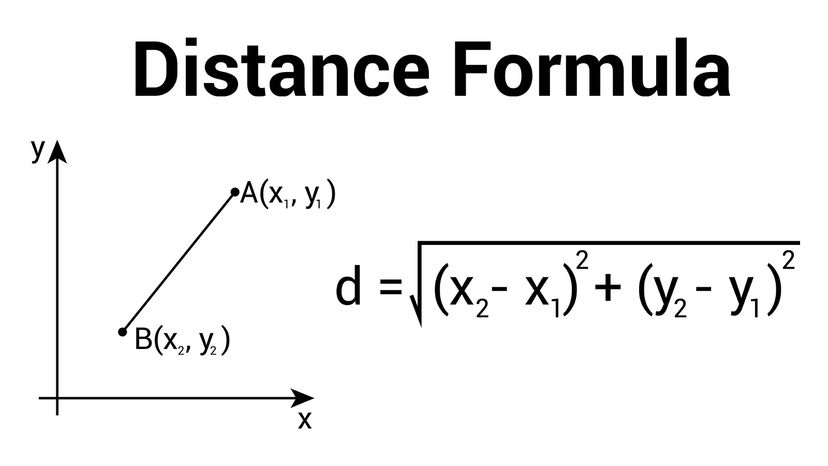# What Is the Formula for Distance?

By: Mark Mancini  |The distance formula is an algebraic expression that gives the shortest distance between two points in a two-dimensional space. Dream01/Shutterstock/HowStuffWorks

You're sitting in math class trying to survive your latest pop quiz. The questions on Page 1 weren't too hard, but on the second page, you see a graph with two little dots on it labeled "Point 1" and "Point 2." And they're connected together by a diagonal line.

Don't panic — you don't even need a distance calculator to tackle this. The distance formula you're looking for is fairly straightforward and has ties to one of the most useful and famous concepts in all of mathematics: the Pythagorean theorem.

Contents

## The Pythagorean Theorem Is Related to Distance Formula

The Pythagorean theorem was named for the Greek philosopher Pythagoras. But he can't take sole credit for discovering it. Old Pythagoras lived from about 570 to 490 B.C.E. Yet more than 1,000 years before he was born, the ancient Babylonians were already aware of the geometric principle that now bears his name.

For those in need of a quick refresher, the Pythagorean theorem says:

The area of the square built upon the hypotenuse of a right triangle is equal to the sum of the areas of the squares upon the remaining sides.

We've got a couple of things to unpack here. A right triangle, also known as a right-angled triangle, is one that contains one 90-degree angle, also known as a right angle. The longest line on a right triangle is called the hypotenuse. (This is the line situated on the opposite side of the right angle.)

Now as we all know, a triangle may have three sides, but a square's got four.

So imagine taking the hypotenuse of a right triangle and turning it into one of the four lines of a brand-new square. Then do the same thing to the other two sides in the original triangle. You'll end up with three individual squares.

As the Pythagorean theorem points out, the square you just made with the hypotenuse will have the same area as the other two squares put together. If the hypotenuse was labeled "c" and those other line segments were labeled "a" and "b," then we could express that idea like so:The Pythagorean theorem says a2 + b2 = c2. The distance formula is derived by using the Pythagorean theorem. grebeshkovmaxim/Shutterstock

## Distance Formula and the Point Coordinate Plane

When most people hear the word "graph," they're picturing a chart with two lines — one vertical, one horizontal — that intersect each other at a right angle.

The vertical line is called the y-axis and its horizontal counterpart is the x-axis. Both lines work together to tell a story with data. Just take a look at this humorous graph from cartoonist Jorge Cham about somebody's not-so-relaxing vacation where the y-axis is labeled "stress" and the x-axis is labeled "time."

In order to make sense of where one point rests on your graph, you need to measure where it falls along the two dimensions (the x-axis and the y-axis). These are known as the point's coordinates. You need to find the coordinates for the first point and the second point before you can calculate the distance between them. You'll use the distance formula to measure the straight line segment connecting the two points.

## How to Derive Distance Formula

Enough preamble. The question you want answered is how to find the distance between two points on a graph (i.e., two sets of two coordinates).

The first point and second point on your graph will each have an x coordinate and a y coordinate. You can calculate the shortest distance between these two points by using the Euclidean distance formula, which is a Pythagorean theorem-related algebraic expression. Here it is, folks:

D = √(x2-x1)2 + (y2-y1)2

Note that "D" means "distance." As for x2 and x1, they refer to the x coordinates of Point 2 and Point 1, respectively. Same goes for y2 and y1, except those are the two y coordinates.

So to calculate the distance, our first step is to subtract x1 from x2. Then we have to multiply the resulting number by itself (or, in other words, "square" that number). After that, we must subtract y1 from y2 and then square the answer we get from doing so.

This will leave us with two numbers we must add together. Then finally, take that number and find its square root. And that square root, ladies and gentlemen, is our distance.

## Calculating the Distance Between Two Points

OK, so let's say Point 1 has an x coordinate of 2 and a y coordinate of 5. Let us also assume that Point 2's got an x coordinate of 9 and a y coordinate of 13.

Plug those values into the handy dandy formula and you get this:

D = √(9-2)2 + (13-5)2

What's 9 minus 2? Easy, 7. And 13 minus 5 is 8, of course.

So now we're left with this:

D = √72 + 82

If you "square" 7 — as in, multiply the number by itself — you end up with 49. As for 8 squared that works out to 64. Let's plug those values into the equation, eh?

D = √49 + 64

Now we're cooking. Add 49 and 64 and you get 113.

D = √113

What's the square root of 113? The answer is 10.63, so therefore:

D = 10.63

Go forth and ace that pop quiz!